Advertisement.

EnchantedLearning.com is a user-supported site.
As a bonus, site members have access to a banner-ad-free version of the site, with print-friendly pages.
Click here to learn more.

(Already a member? Click here.)

 You might also like: Generate Your Own Subtraction Worksheets Generate Subtraction Worksheets: One Digit + One Digit, Vertical Format Generate Subtraction Worksheets: One Digit + One Digit Subtract Groups of Pets Worksheet Printout Subtract Groups of Flowers Worksheet Printout Today's featured page: Name Theme Page

 Our subscribers' grade-level estimate for this page: 2nd - 3rd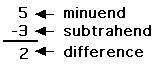More Math Activities
 EnchantedLearning.comSubtraction Worksheets Back to the Subtraction PageSubtracting Decimals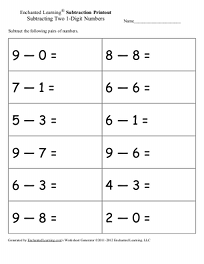Generate Printable Subtraction Worksheets:One Digit - One Digit + One DigitYou can generate printable subtraction worksheets (one page of questions and one page of answers) with 8, 10 or 12 problems. Every time you click, you get a new worksheet, with a new set of questions and answers. For subscribers only.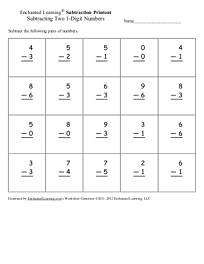Generate Printable Subtraction Worksheets:One Digit - One Digit, Vertical FormatYou can generate printable subtraction worksheets (one page of questions and one page of answers) with 16 or 20 problems. Every time you click, you get a new worksheet, with a new set of questions and answers. For subscribers only.

Worksheets: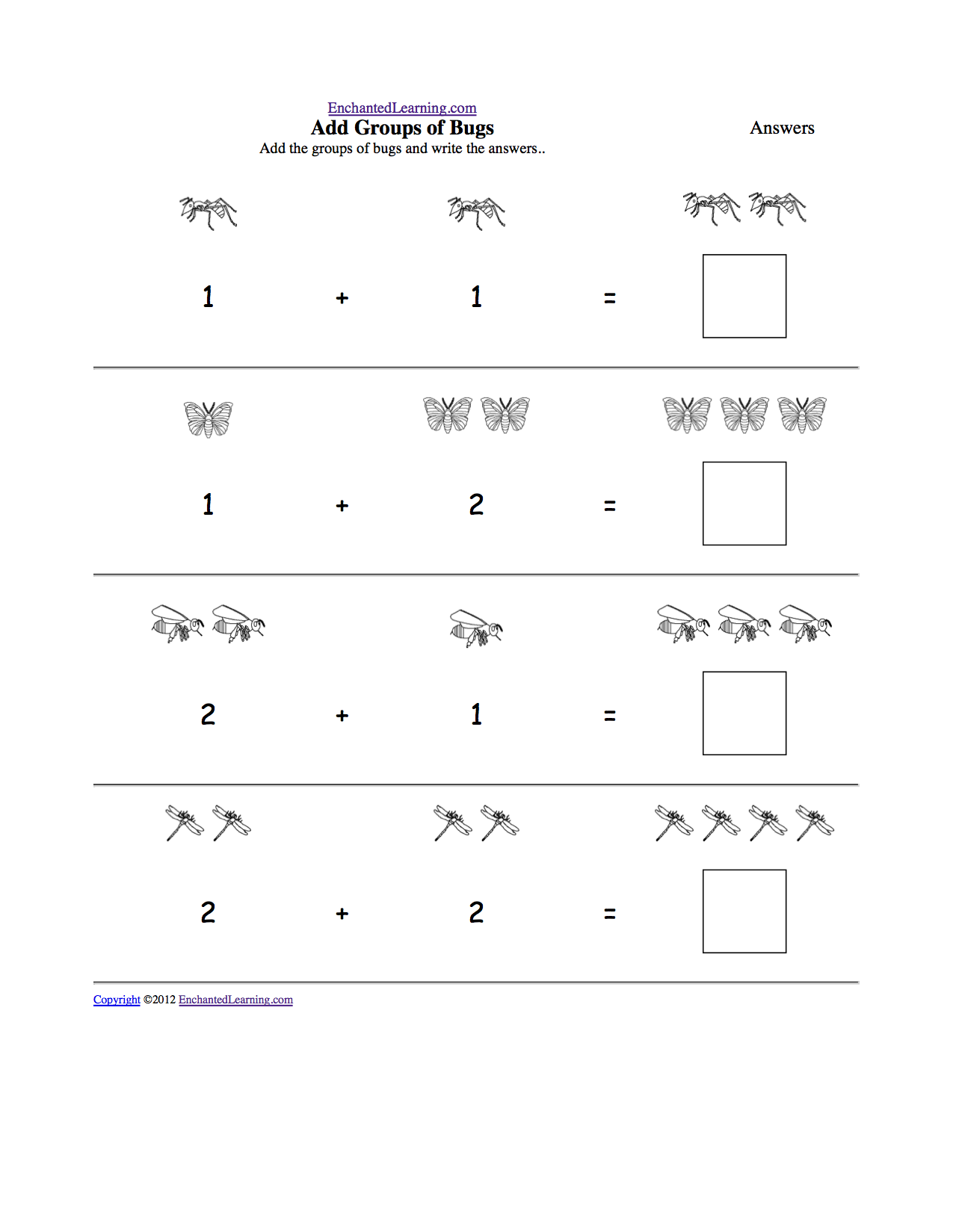Visual Subtraction: Subtract Groups of ItemsPrintable Worksheet Subtract the groups of common items and write the answers. Subtract bugs or the answers, Subtract flowers or the answers Subtract food or the answers Subtract ocean animals or the answers Subtract pets or the answers.Printable Set of Subtraction DominoesPrint a set of subtraction dominoes; each tile has subtraction problems on it (for example, 7 - 5). The numbers from zero to ten are used in the subtraction problems and the answers are the numbers from zero to six. In this game the student matches the answers of subtraction problems.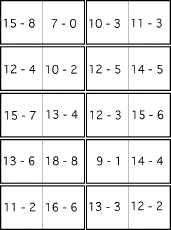Printable Set of Subtraction Dominoes #2Print a set of subtraction dominoes; each tile has subtraction problems on it (for example, 16 - 7). The numbers from one to twenty-two are used in the subtraction problems and the answers are the numbers from seven to thirteen. In this game the student matches the answers of subtraction problems.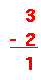1 Digit Minus 1 DigitSubtract a one-digit number from another one-digit number - vertical orientation.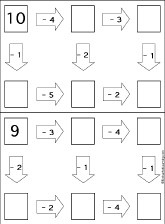Subtraction Follow-the-Arrows Puzzles Do subtraction problems along the paths according to the arrows until you get to the end. You should get to the same final answer three different ways. Puzzle #1, or the answers Puzzle #2, or the answers Puzzle #3, or the answers Puzzle #4, or the answers Puzzle #5, or the answersWord Problems: Subtracting 2 NumbersDraw a picture or use an equation to help you solve each subtraction problem.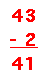2 Digits Minus 1 DigitSubtract a one-digit number from a two-digit number - vertical orientation.3 Digits Minus 1 DigitSubtract a one-digit number from a three-digit number - vertical orientation.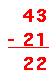2 Digits Minus 2 DigitsSubtract a two-digit number from a two-digit number - vertical orientation.3 Digits Minus 2 DigitsSubtract a two-digit number from a three-digit number - vertical orientation.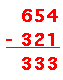3 Digits Minus 3 DigitsSubtract a three-digit number from a three-digit number - vertical orientation.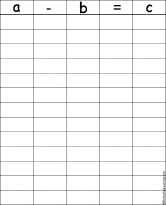Subtraction ChartWrite many equations in which a-b=c, like 3-1=2.Valentine's Day:Subtract, then Decode the RiddleSolve the subtraction problems, then use the alphabet code to decode the Valentine's Day riddle, "What do you call a very small valentine?" Answer - A valentiny. Or go to the answers.Many Ways of Expressing NumbersMath Graphic Organizers10 graphic organizers to use to express numbers in different ways, from "2 Ways to Make 2" to "10 Ways to Make 10."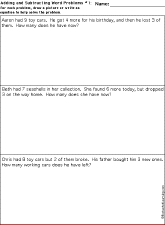Word Problems: Adding and Subtracting NumbersDraw a picture or use an equation to help you solve each subtraction problem. Worksheet #1 Answers: 9-4+3=8, 7+6-3=10, 8-2+3=9. Worksheet #2 Answers: 13-6+4=11, 15-4+5=16, 10-3+5=12. Worksheet #3 Answers: 20-4+3=19, 7+6-3=10, 9-2+3=10. Worksheet #4 Answers: 32+11-3=40, 15-4+8=19, 14-5+8=17. Worksheet #5 Answers: 32-6+4=30, 8+3-3=8, 13-2+5=16. Worksheet #6 Answers: 15-4+6=17, 25-6+8=27, 19-4+3=18. Worksheet #7 Answers: 28-9+5=24, 8+5-4=9, 16-4+6=18. Worksheet #8 Answers: 24-6+9=27, 21-9+3=15, 31-2+13=42. Subtracting Decimals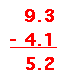Each worksheet has decimal numbers to subtract. Subtracting Fractions With the Same DenominatorsSubtract the fractions (they have the same denominators). Subtracting Fractions With Different DenominatorsSubtract the fractions (they have different denominators). Subtracting Fractions Word Problems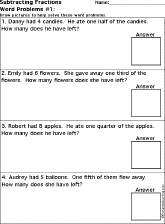Solve word problems that involve subtracting fractions. Draw pictures to help solve these word problems.

In the following activities, the student does simple math problems and then letter substitutions to answer an animal-related question. Click to go to a printable resource.

 Dinosaur-related Puzzles Subtraction of two single-digit numbers: Insect-related PuzzlesSubtraction of two single-digit numbers: Whale-related PuzzlesSubtraction of two single-digit numbers:

Printable Subtraction Books: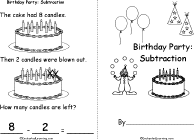Birthday Party: SubtractionPrintable BookA short, printable book about subtracting birthday party items, including candles, ice cream cones, balloons, party hats, birthday cards, gifts, juggling balls, lollipops, and candies.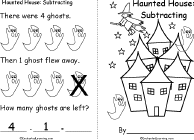Haunted House: SubtractionPrintable BookA short, printable book about subtracting Halloween items, including ghosts, witches, bats, skulls, spiders, webs, cats, skeletons and jack-o'-lanterns.Pumpkin Patch: SubtractingPrintable BookA short, printable book about subtracting fall items, including pumpkins, scarecrows, apples, ears of corn, webs, leaves, pies and haystacks.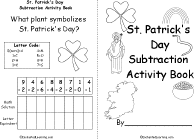St. Patrick's Day Subtraction Activity BookA St. Patrick's Day subtraction activity book in which the readers solves a series of subtraction problems and then does a letter substitution in order to answer St. Patrick's Day questions.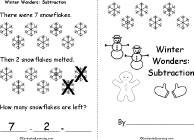Winter Wonders: SubtractionPrintable BookA short, printable book about subtracting winter items, including snowflakes, snowmen, sleds, mittens, evergreen trees, scarves, earmuffs, caps, and skates.

Subtracting US Coins:Subtracting PenniesSubtract the pennies in these printouts:Subtracting Pennies: Printout #1Subtracting Pennies: Printout #2Subtracting Pennies: Printout #3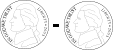Subtracting NickelsSubtract the nickels in these printout:Subtracting Nickels: Printout #1Subtracting Nickels: Printout #2Subtracting Nickels: Printout #3Subtracting DimesSubtract the dimes in these printouts:Subtracting Dimes #1Subtracting Dimes #2Subtracting Dimes #3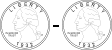Subtracting QuartersSubtract the quarters in these printouts:Subtracting Quarters #1Subtracting Quarters #2Subtracting Quarters #3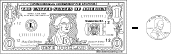US Dollar Minus Pennies (Printable)Subtract pennies from a dollar.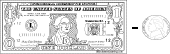US Dollar Minus Nickels (Printable)Subtract nickels from a dollar.US Dollar Minus Dimes (Printable)Subtract dimes from a dollar.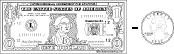US Dollar Minus Quarters (Printable)Subtract quarters from a dollar.

St. Patrick's Day Subtraction Activities:St. Patrick's Day Subtraction Activity BookA St. Patrick's Day subtraction activity book in which the readers solves a series of subtraction problems and then does a letter substitution in order to answer St. Patrick's Day questions.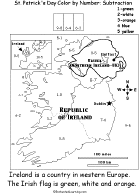St. Patrick's Day Color by Number Using SubtractionColor St. Patrick's Day pictures by solving by solving simple subtraction problems and then coloring by number (Printout #1, Printout #2, Printout #3, Printout #4). For a pdf version of the four subtraction-color by number pages, click here (site members only).

Enchanted Learning®
Over 35,000 Web Pages
Sample Pages for Prospective Subscribers, or click below

 Overview of Site What's New Enchanted Learning Home Monthly Activity Calendar Books to Print Site Index K-3 Crafts K-3 Themes Little ExplorersPicture dictionary PreK/K Activities Rebus Rhymes Stories Writing Cloze Activities Essay Topics Newspaper Writing Activities Parts of Speech Fiction The Test of Time iPhone app TapQuiz Maps - free iPhone Geography Game Biology Animal Printouts Biology Label Printouts Biomes Birds Butterflies Dinosaurs Food Chain Human Anatomy Mammals Plants Rainforests Sharks Whales Physical Sciences: K-12 Astronomy The Earth Geology Hurricanes Landforms Oceans Tsunami Volcano Languages Dutch French German Italian Japanese (Romaji) Portuguese Spanish Swedish Geography/History Explorers Flags Geography Inventors US History Other Topics Art and Artists Calendars College Finder Crafts Graphic Organizers Label Me! Printouts Math Music Word Wheels

Click to read our Privacy Policy

## Enchanted Learning Search

 Search the Enchanted Learning website for:

Advertisement.

Advertisement.

Advertisement.

Copyright ©2004-2018 EnchantedLearning.com ------ How to cite a web page